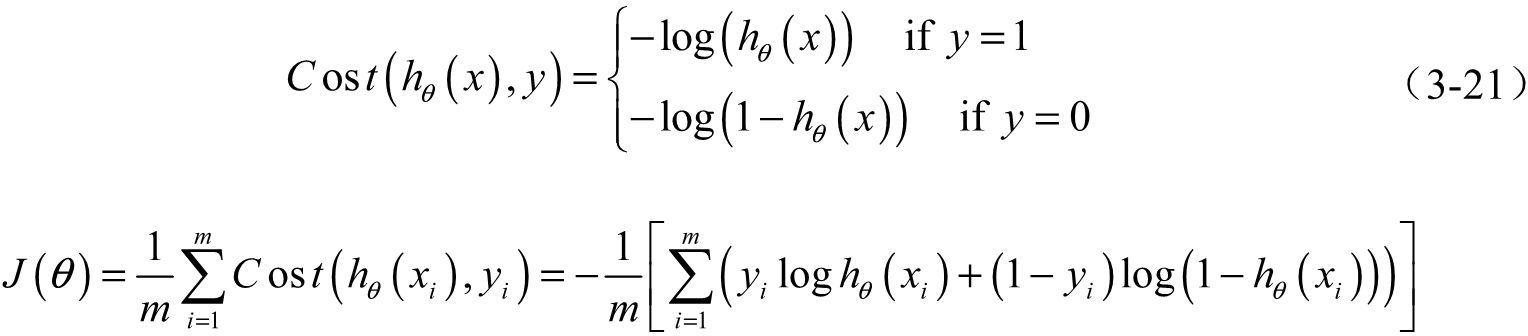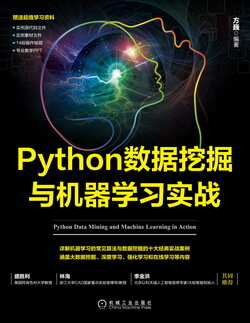# Python 数据挖掘与机器学习实战 (71)：回归分析介 3.6.2(构造损失函数 J)

$p\left(y|x;\theta \right)=\left({h}_{\theta }\left(x\right){\right)}^{y}\left(1-{h}_{\theta }\left(x\right){\right)}^{1-y}\phantom{\rule{1em}{0ex}}\phantom{\rule{1em}{0ex}}\phantom{\rule{1em}{0ex}}\phantom{\rule{1em}{0ex}}\phantom{\rule{1em}{0ex}}\phantom{\rule{1em}{0ex}}\phantom{\rule{1em}{0ex}}\left(3-17\right)$$p(y|x;\theta)=(h_{\theta}(x))^y(1-h_{\theta}(x))^{1-y}\quad\quad\quad\quad\quad\quad\quad(3-17)$

$L\left(\theta \right)=\prod _{i=1}^{m}P\left({y}_{i}|{x}_{i};\theta \right)=\prod _{i=1}^{m}\left({h}_{\theta }\left({x}_{i}\right){\right)}^{{y}_{i}}\left(1-{h}_{\theta }\left({x}_{i}\right){\right)}^{1-{y}_{i}}\phantom{\rule{1em}{0ex}}\phantom{\rule{1em}{0ex}}\phantom{\rule{1em}{0ex}}\phantom{\rule{1em}{0ex}}\phantom{\rule{1em}{0ex}}\phantom{\rule{1em}{0ex}}\phantom{\rule{1em}{0ex}}\left(3-18\right)$$L(\theta)=\prod\limits^m_{i=1}P(y_i|x_i;\theta)=\prod\limits^m_{i=1}(h_{\theta}(x_i))^{y_i}(1-h_{\theta}(x_i))^{1-y_i}\quad\quad\quad\quad\quad\quad\quad(3-18)$

$l\left(\theta \right)=\mathrm{log}L\left(\theta \right)=\sum _{i=1}^{m}\left({y}_{i}\mathrm{log}{h}_{\theta }\left({x}_{i}\right)+\left(1-{y}_{i}\right)\mathrm{log}\left(1-{h}_{\theta }\left({x}_{i}\right)\right)\right)\phantom{\rule{1em}{0ex}}\phantom{\rule{1em}{0ex}}\phantom{\rule{1em}{0ex}}\phantom{\rule{1em}{0ex}}\phantom{\rule{1em}{0ex}}\phantom{\rule{1em}{0ex}}\phantom{\rule{1em}{0ex}}\left(3-19\right)$$l(\theta)=\log L(\theta)=\sum\limits^m_{i=1}(y_i\log h_{\theta}(x_i)+(1-y_i)\log(1-h_{\theta}(x_i)))\quad\quad\quad\quad\quad\quad\quad(3-19)$

$J\left(\theta \right)=-\frac{1}{m}l\left(\theta \right)\phantom{\rule{1em}{0ex}}\phantom{\rule{1em}{0ex}}\phantom{\rule{1em}{0ex}}\phantom{\rule{1em}{0ex}}\phantom{\rule{1em}{0ex}}\phantom{\rule{1em}{0ex}}\phantom{\rule{1em}{0ex}}\left(3-20\right)$$J(\theta)=-\frac{1}{m}l(\theta)\quad\quad\quad\quad\quad\quad\quad(3-20)$